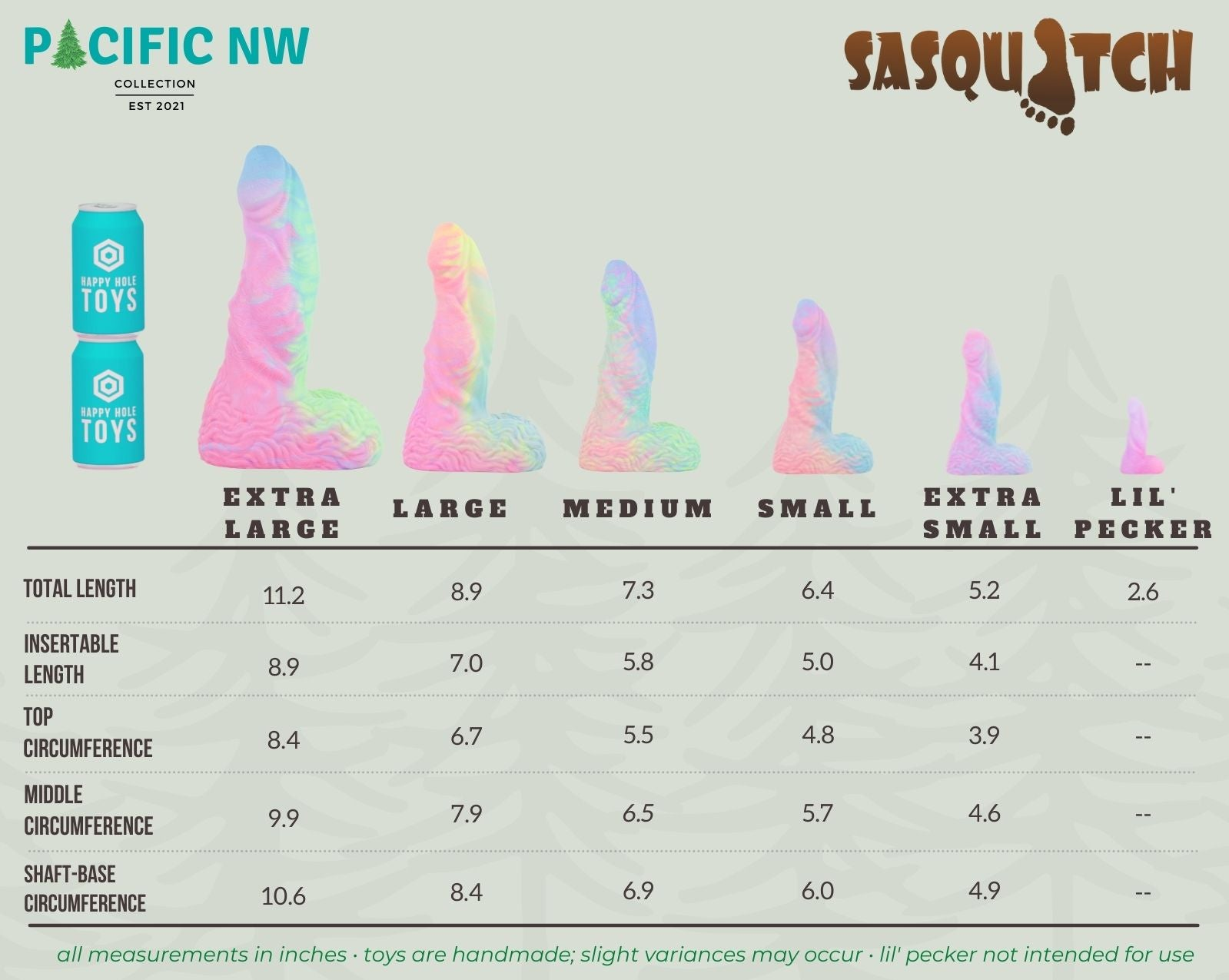# Sasquatch Size Chart

Text-reader friendly Sasquatch size information can be found after this image:OCR-friendly Sasquatch size information:

• Sasquatch XSmall: Total Length is 5.2 inches and the Insertable Length is 4.1 inches. The Top Circumference is 3.9 inches, the Middle Circumference is 4.6 inches, and the Shaft-Base Circumference is 4.9 inches
• Sasquatch Small: Total Length is 6.4 inches and the Insertable Length is 5.0 inches. The Top Circumference is 4.8 inches, the Middle Circumference is 5.7 inches, and the Shaft-Base Circumference is 6.0 inches.
• Sasquatch Medium: Total Length is 7.3 inches and the Insertable Length is 5.8 inches. The Top Circumference is 5.5 inches, the Middle Circumference is 6.5 inches, and the Shaft-Base Circumference is 6.9 inches.
• Sasquatch Large: Total Length is 8.9 inches and the Insertable Length is 7.0 inches. The Top Circumference is 6.7 inches, the Middle Circumference is 7.9 inches, and the Shaft-Base Circumference is 8.4 inches.
• Sasquatch XLarge: Total Length is 11.2 inches and the Insertable Length is 8.9 inches. The Top Circumference is 8.4 inches, the Middle Circumference is 9.9 inches, and the Shaft-Base Circumference is 10.6 inches.